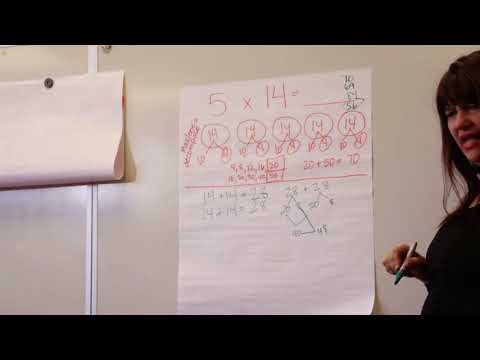# Properties and patterns for multiplication (Full video)

Description: Sal changes the order of numbers or decomposes numbers to simplify multiplication problems. Created by Sal Khan. And the reason why it's useful to not just always have to count it, but to be able to use little multiplication with the number of rows and the number of columns is that you might run into things and you will run into things where it's very hard to count each of the objects individually, but it might be a little bit easier to count the rows and to count the columns. So you could view this 4 as an array of objects where we have 4 rows. And you might already remember that we can calculate the total number of objects by multiplying the rows times the columns.

### Other videos you might be interested in### Using Properties of Operations to Multiply (Hofmayer) (Full video)

#### Teaching the Core